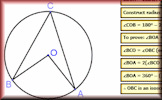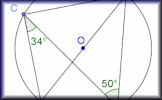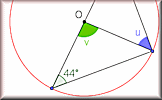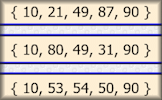#Click on a picture above for a large version and interactive model or show a theorem at Random.

#### Proof of Circle TheoremsArrange the stages of the proofs for the standard circle theorems in the correct order with this drag-and-drop activity.

The short web address is:

Transum.org/go/?to=provetheorem

#### Circle Theorem PairsA pairs game based around ten theorems about the angles made with chords, radii and tangents of circles.

The short web address is:

Transum.org/go/?to=circletheorempairs

#### Circle Theorem WorksheetOne page of ready-drawn circles for pupils to draw their own diagrams followed by two pages of questions.

The short web address is:

Transum.org/go/?to=circletheoremworksheet

#### Circle Theorems ExerciseShow that you understand the circle theorems with this self marking exercise of traditional circle angle questions.

The short web address is:

Transum.org/go/?to=circleangles

There are also Circle Theorems Pairs games, a Circle Theorems Worksheet and a Circle Theorems Online Exercise available.

#### Great ExpectationAn interactive online activity requiring logical thinking and a certain amount of luck. Numbers 1 to 6 are presented randomly and are to be used to produce two 2-digit numbers. Can you ensure that the first number is greater than the second?

This is a Shine+Write visual aid designed to be projected onto a whiteboard for whole class exposition.

You can find other popular visual aids on our Shine+Write page.

Find out about the latests developments on this website by signing up for a Transum Subscription. You will receive a regular newsletter and have access to the answers to most of the puzzles and activities on this site.

Do you have any comments? It is always useful to receive feedback and helps make this free resource even more useful for those learning Mathematics anywhere in the world. Click here to enter your comments.

#### Standard DeviationFind how 'spread out' data is by estimating and calculating this measure of dispersion. So far this activity has been accessed 108 times and 5 people have earned a Transum Trophy for completing it.For All:

©1997-2019 WWW.TRANSUM.ORG